# Examples for secondary school students

#### Number of problems found: 2059

• Derivation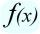Exists a function whose derivation is the same function?
• RootsDetermine the quadratic equation absolute coefficient q, that the equation has a real double root and the root x calculate: ?
• Reciprocal equation 2Solve this equation: x + 5/x - 6 = 4/11
• Missing termWhat is the missing term in the sequence? _____, 13, 30, and 47?
• Log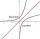Calculate value of expression log |3 +7i +5i2| .
• AP - simpleFind the first ten members of the sequence if a11 = 132, d = 3.
• DiceWe throw five times the dice. What is the probability that six fits exactly twice?
• AP - basicsDetermine first member and differentiate of the the following sequence: a3-a5=24 a4-2a5=61
• Terms of GPWhat is the 6th term of the GP 9, 81, 729,. .. ?
• Scalene triangleSolve the triangle: A = 50°, b = 13, c = 6
• Negative difference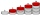Find four arithmetic progression members between 7 and -6.
• Number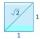Is number 5.146852 irrational?
• Expressions 3If k(x+6)= 4x2 + 20, what is k(10)=?
• Simple interest 3Find the simple interest if 11928 USD at 2% for 10 weeks.
• Committees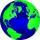How many different committees of 2 people can be formed from a class of 21 students?
• Permutations without repetitionFrom how many elements, we can create 720 permutations without repetition?Given that P = (5, 8) and Q = (6, 9), find the component form and magnitude of vector PQ.
• Sequence - 5 membersWrite first five members of the sequence ?
• The observatoryThe dome of the hemisphere-shaped observatory is 5.4 meters high. How many square meters of sheet metal needs to be covered to cover it, and 15 percent must be added to the minimum amount due to joints and waste?
• Isosceles trapezoidCalculate the content of an isosceles trapezoid whose bases are at ratio 5:3, the arm is 6cm long and it is 4cm high.

Do you have an interesting mathematical word problem that you can't solve it? Submit a math problem, and we can try to solve it.

We will send a solution to your e-mail address. Solved examples are also published here. Please enter the e-mail correctly and check whether you don't have a full mailbox.

Please do not submit problems from current active competitions such as Mathematical Olympiad, correspondence seminars etc...